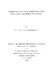# A production function analysis of fresh milk production in the highlands of Eritrea.2004

## Abstract

This study presents a production function analysis of fresh milk producers in the Highlands of Eritrea for the year 2002, dealing with the most important factors of production. Most dairy farmers are located in the Central Zone and Southern Zone (Mendefera and Dekemhare) areas of the Highlands of Eritrea. To ensure representative production functions, the Highlands of Eritrea were divided into three respectively homogenous study areas, namely Central Zone, Mendefera and Dekemhare. Most data for this study were collected by survey using a questionnaire, as dairy farms' recorded data were scarce. The annual milk yield record and purchased concentrates per farmer were obtained from their respective milk collecting centres and Dairy Associations belonging to each study area. Basically, an attempt was made to pool the data of the three study areas, using dummy variables to test if the three study areas' regressions have a common intercept and a common slope. However, from the analysis, the intercept and slope dummy coefficients for the pooled data were found to be statistically significant at the 1 % and 5% levels of probability. Thus, it was not economically as well as statistically practical to pool the three areas' data to determine a common function that represents the sample dairy farmers of the Highlands of Eritrea as a whole. For this reason, a separate analysis was conducted for each study area. The analysis used the Cobb-Douglas function (double-log) form using multiple regressions. However, while analysing the data using ordinary least squares (OLS) regressions, strong intercorrelations were encountered among some factors of production. These intercorrelations resulted in some of the parameters having negative production coefficients where, a priori, all such coefficients are assumed to be non-negative. Thus, to tackle the multicollinearity problem, a ridge regression technique was used at different levels of the biasing constant, c, where the regression coefficients in the ridge trace start to stabilize and the variance inflation factor (VIF) of each parameter and the average of the VIFs are close to one. The final fitted model includes those variables, which were significant at the 1 % and 5% levels of probability. However, for the Mendefera study area those variables significant at 10% level of probability were included as their t-statistic values were considerably greater than one and nearly significant at the 5% level of probability. From the regression coefficients of the final fitted model for each study area, the elasticities of production with respect to the factors of production, ceteris paribus, were estimated. The highest response in production to a one percent change, ceteris paribus, is due to milking cows followed by concentrates and labour for the Central Zone Dairy farmers . However, for the Southern Zone (Mendefera and Dekemhare) the highest response next to milking cows came from forage and labour. The regression coefficients of all the factors of production in each study area were greater than zero and less than one, implying rational use of the resources. However, the sum of the elasticities of production was found to be greater than one for each area of production, indicating increasing returns to scale. Components of the production function and cost calculations including marginal product (MP), values of marginal product (VMP), marginal rate of substitution (MRS), least-cost combinations of inputs, profit maximizing combinations of inputs and the short-run cost functions for each category within the sample of dairy farmers in each study area were estimated. All the VMP's of the resources for the Central Zone dairy farmers were found to be greater than the corresponding unit price of the resources. This implies that the resources are utilized inadequately. However, for the Southern Zone (Mendefera and Dekemhare) the variable concentrates is over-utilized, as the VMP is less than the unit price of the input. The marginal rate of substitution of concentrates for forage, ceteris paribus, showed that the Central Zone sample dairy farmers were utilizing the two resources almost equally. But for the Southern Zone sample dairy farmers the MRS of the mentioned resources showed a higher dependence on concentrates than forage. From the least-cost combination of concentrates and forage analysis it was found that none of the sample of dairy farmers was allocating resources on a least-cost basis. The profit maximizing combination of inputs showed generally a considerable improvement of milk yield and margins for all the sample of dairy farmers relative to the present situations. However, the profit maximizing criteria (i.e. VMPx = Px), assumes perfect knowledge, a risk free environment and competitive marketing systems. This has to be considered when advising sample farmers as to the optimal combination of concentrates and forage. The short-run cost function also indicates use of resources at below optimum levels. When the average variable cost of the resources is less than the unit price of output, then use of the resources is in the rational area of production. Based on the analysis of the three study areas, the average variable cost of the lower one-third group of sample dairy farmers of the Southern Zone was found to be greater than the unit price of output. This means that the farmers were not covering the short-run costs of production. The MC of concentrates for the lower one-third group of sample dairy farmers was found to be greater than the price per litre of fresh milk in the Southern Zone. This implies more than optimum use of the input (i.e. where MC = Py).

## Description

Thesis (M.Sc.)-University of KwaZulu-Natal, Pietermaritzburg, 2004.

## Keywords

Milk--Economic aspects--Eritrea., Milk yield--Eritrea., Dairy farming--Eritrea., Theses--Animal and poultry science.